EnchantedLearning.com is a user-supported site.
As a bonus, site members have access to a banner-ad-free version of the site, with print-friendly pages.

 Math EnchantedLearning.comSymmetry Shapes

 Lines of SymmetryThis crescent has one line of symmetry.This diamond has two lines of symmetry.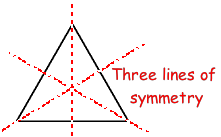This triangle has three lines of symmetry.

A figure exhibits symmetry when part of the figure is the mirror image of another part of the figure. For example, an object has line (or reflection) symmetry when one side of the figure is a mirror image of the other across the line of symmetry (for example, a heart has linear symmetry). An object has plane symmetry when two halves of the object are mirror reflections of each other across a plane of symmetry (for example, a cylinder has plane symmetry). An object has radial (or point) symmetry when it is symmetric around a point (for example, a circle has point symmetry since every point on the circle has a mirror image of itself across the central point).

Printable Worksheets: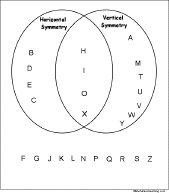Capital Letter Symmetry Venn DiagramUse a Venn diagram to classify capital letters by their symmetry, whether they have horizontal symmetry, vertical symmetry, both types, or neither. Or go to the answers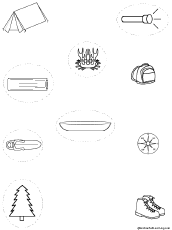Circle Symmetrical PicturesOn each of these printable worksheets, the student circles the pictures that are symmetrical.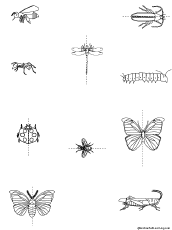Draw Lines of SymmetryOn each of these printable worksheets, the student draws the lines of symmetry, if they exist.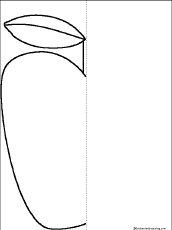Finish the Symmetrical Picture WorksheetsOn each of these printable worksheets, the student finishes a drawing around the line of symmetry (horizontal, vertical, or diagonal.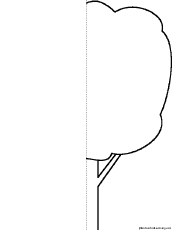Finish the Symmetrical Picture Worksheets and Fill in the Missing LettersOn each of these printable worksheets, the student finishes a drawing around the line of symmetry (horizontal, vertical, or diagonal. Then the students fills in missing letters in the word(s) that describes the picture.Finish Four Symmetrical Pictures WorksheetsOn each of these printable worksheets, the student finishes four drawings around the line of symmetry (horizontal, vertical, or diagonal.

## Enchanted Learning Search

 Search the Enchanted Learning website for: